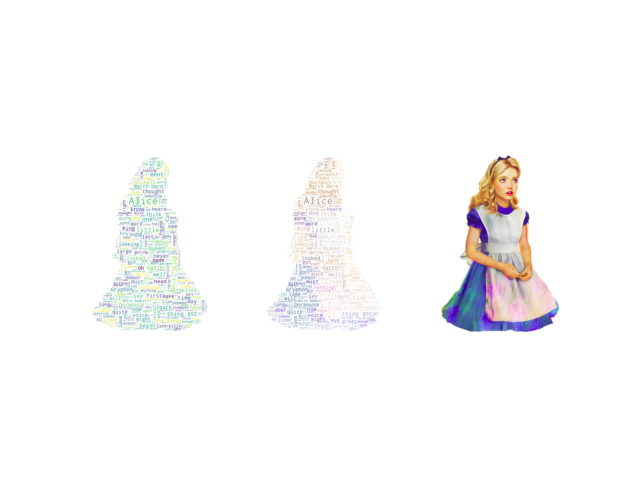# Image-colored wordcloud¶

You can color a word-cloud by using an image-based coloring strategy implemented in ImageColorGenerator. It uses the average color of the region occupied by the word in a source image. You can combine this with masking - pure-white will be interpreted as ‘don’t occupy’ by the WordCloud object when passed as mask. If you want white as a legal color, you can just pass a different image to “mask”, but make sure the image shapes line up.```from os import path
from PIL import Image
import numpy as np
import matplotlib.pyplot as plt
import os

from wordcloud import WordCloud, STOPWORDS, ImageColorGenerator

# get data directory (using getcwd() is needed to support running example in generated IPython notebook)
d = path.dirname(__file__) if "__file__" in locals() else os.getcwd()

# Read the whole text.
text = open(path.join(d, 'alice.txt')).read()

# read the mask / color image taken from
# http://jirkavinse.deviantart.com/art/quot-Real-Life-quot-Alice-282261010
alice_coloring = np.array(Image.open(path.join(d, "alice_color.png")))
stopwords = set(STOPWORDS)

wc = WordCloud(background_color="white", max_words=2000, mask=alice_coloring,
stopwords=stopwords, max_font_size=40, random_state=42)
# generate word cloud
wc.generate(text)

# create coloring from image
image_colors = ImageColorGenerator(alice_coloring)

# show
fig, axes = plt.subplots(1, 3)
axes.imshow(wc, interpolation="bilinear")
# recolor wordcloud and show
# we could also give color_func=image_colors directly in the constructor
axes.imshow(wc.recolor(color_func=image_colors), interpolation="bilinear")
axes.imshow(alice_coloring, cmap=plt.cm.gray, interpolation="bilinear")
for ax in axes:
ax.set_axis_off()
plt.show()
```

Total running time of the script: ( 0 minutes 2.209 seconds)

Gallery generated by Sphinx-Gallery